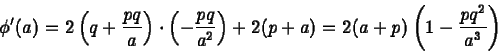# Algebraic steps to simplify an equation

• JOhnJDC

## Homework Statement

Could someone show me the algebraic steps involved in the following equivalency?

## Homework Equations## The Attempt at a Solution

EDIT: see attempt below. I eventually answered my own question. Thanks, Mark44.

Last edited:

## Homework Statement

Could someone show me the algebraic steps involved in the following equivalency?

## Homework Equations## The Attempt at a Solution

I get lost in the algebra every time.

$$2(q + \frac{pq}{a})(\frac{-rq}{a^2}) + 2(p + a)$$
$$=2(\frac{-pq^2}{a^2} + \frac{-p^2q^2}{a^3}) + 2(p + a)$$

Get a common denominator for the two terms in the first pair of parentheses, and then combine those two terms.
Can you carry on from there?

$$2(q + \frac{pq}{a})(\frac{-rq}{a^2}) + 2(p + a)$$
$$=2(\frac{-pq^2}{a^2} + \frac{-p^2q^2}{a^3}) + 2(p + a)$$

Get a common denominator for the two terms in the first pair of parentheses, and then combine those two terms.
Can you carry on from there?

Okay, the two terms in parentheses now have a common denominator:

2(a+p) + 2(-apq^2 - p^2q^2)/a^3

This is where I get stuck. I don't know how to factor 2(a+p) out of the numerator 2(-apq^2 - p^2q^2).

Here is my attempt:

2a+2p + (-2apq^2 - 2p^2q^2)/a^3

(2a+2p)[1 + (-pq^2 - pq^2)/a^3]

2(a+p)[1 + (-2pq^2)/a^3]

What am I doing wrong? Why do I end up with -2pq^2/a^3 instead of just pq^2/a^3? Thanks.

EDIT: I got it. I was thinking about the operation in the wrong way. Instead of trying to factor out (a+p), I decided to factor out -pq^2 from 2(-apq^2-p^2q^2)/a^3, which yielded 2[-pq^2(a+p)/a^3]. From there, I could easily factor out 2(a+p) from 2(a+p) + 2(-apq^2 - p^2q^2)/a^3.

Thanks.

Last edited: